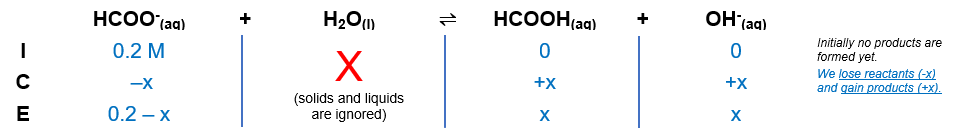# Problem: 25.0 mL of 0.2 M sodium formate was titrated with 0.1 M HCl. What is the pH: a) before HCl is added; b) at the half-equivalence point; and c) at the equivalence point? Possible answers, respectively, are given below.A. 8.52; 3.75; 2.46B. 7.52; 4.75; 3.46C. 6.52; 5.75; 5.46D. 5.52; 2.75; 1.46E. 9.52; 6.75; 4.46

###### FREE Expert Solution

We're being asked to calculate the pH a) before HCl is added; b) at the half-equivalence point; and c) at the equivalence point.

NaHCOO (sodium formate) will break up in the solution:

NaHCOO(aq) → Na+(aq) + HCOO-(aq)

• HCOO- is the conjugate base of HCOOH → HCOO- is a weak base

HCOO- weak baseproton acceptor
H2O → will act as the weak acidproton donor

Equilibrium reaction:        HCOO-(aq) + H2O(l)  HCOOH(aq) + OH-(aq)

Step 1: Construct an ICE chart for the reaction.84% (325 ratings)###### Problem Details

25.0 mL of 0.2 M sodium formate was titrated with 0.1 M HCl. What is the pH: a) before HCl is added; b) at the half-equivalence point; and c) at the equivalence point? Possible answers, respectively, are given below.

A. 8.52; 3.75; 2.46

B. 7.52; 4.75; 3.46

C. 6.52; 5.75; 5.46

D. 5.52; 2.75; 1.46

E. 9.52; 6.75; 4.46